PHYSICS

Mass

WAVE AND PHOTON

WA. Fundamental of Wave WB. Photon WC. Kong Vector
WE. Behaviors of Photon

WC. KONG VECTOR

1. Structure of Photon 2. Kong Vector

INTRODUCTION

This chapter introduces the structure of photon and the Kong vector. The Kong vector is the velocity of photon. This chapter discusses the basis of the characteristic of photon. This basis is the reason for the various behaviors of photon.

OBJECTIVES

1)      To describe the structure of photon.

2)      To introduce the Kong vector.

3)   To illustrate the Kong vector of photons under different conditions.

## WC.1.0        STRUCTURE OF PHOTON

Photon possesses magnetic and electric (M&E) characteristics. The M&E components are perpendicular to each other. The M&E components are fixed at each frequency. It is because each component creates each and maintains the existence of each component. The ratio of the electric to the magnetic component at perpendicular position is equal to the speed of light. Photon oscillates in the M&E field on certain frequency depending on the energy level.

Photon has linear momentum. Photon travels in vacuum space at the ultimate speed of light. Photon requires a virtual medium to travel. The virtual medium is the M&E field. Photon is named here as the kinetic-time-energy. It is a kind of energy, transfers from one point to another point, by oscillating through the surrounding M&E field. The oscillation and velocity of photon depends on the pattern of M&E field.

Photon is different from particle wave such as water wave. Photon transfers energy in 3-dimensional while particle wave is only 2-dimensional. The y- and z-component of photon is the M&E components and the x-component is the velocity. For particle wave, the y-component is the magnitude of energy and x-component is the velocity.

WC.2.0        KONG VECTOR

Photon consists of the M&E components. Photon travels at certain velocity, v. These three components form the structure of photon. The structure of photon is illustrated in figure WC.2.1 below.

The electric component is represented by the symbol ‘E’, the magnetic component is represented by the symbol ‘B’ and the velocity of photon is represent by the symbol ‘v’.

The velocity of photon is described by a vector v, called the Kong vector. The Kong vector is defined as

B2v = E x B                                                                                         … eq. WC.2.1

The direction of v at any point gives the direction of travel of the photon at that point. The cross product of the electric field to the magnetic field, E x B, points to the direction in which the photon is traveling.

To solve mathematically, eq. WC.2.1 becomes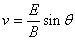… eq. WC.2.2

In the vacuum space, the 3 components are perpendicular; the photon travels at the speed of light,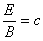… eq. WC.2.3

where   c = speed of light

sin q  = 1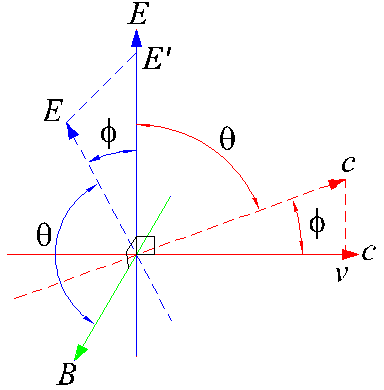Figure WC.2.1 – Vector diagram of photon. E is the electrical component, B is the magnetic component and v is the traveling velocity of photon.

WC.2.1        Velocity of Kong Vector

In cases when the M&E components are not perpendicular, where the electrical component is twisted from the vertical direction, the E becomes E’ as shown in figure WC.2.1.

From eq. WC.2.2, the velocity of photon is… eq. WC.2.2

For 0 < q < 180°... eq. WC.2.4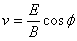… eq. WC.2.5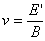... eq. WC.2.6

We substitute eq. WC.2.3 into eq. WC.2.5, the velocity of photon becomes

v = c cos f                                                                                         … eq. WC.2.7

The Kong vector is then reduced. The photon travels at velocity lower than the speed of light. The Kong vector at lower magnitude is shown in figure WC.2.1.

WC.2.2        Reflected Kong Vector

In cases when the M&E components are twisted more than 90° from the vertical axis, which is shown in figure WC.2.2. The component E becomes E’’.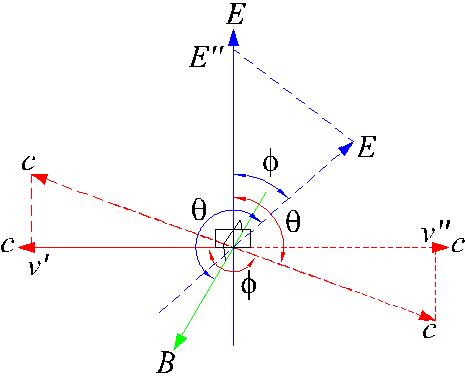Figure WC.2.2 – Vector diagram of photon. E is the electrical component, B is the magnetic component and v’ is the traveling velocity of photon.

From eq. WC.2.2, the velocity of photon is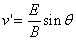… eq. WC.2.2

For 180° < q < 360°, 90° < f  < 270°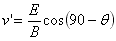... eq. WC.2.8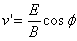… eq. WC.2.9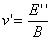... eq. WC.2.10

We substitute eq. WC.2.3 into eq. WC.2.9, the velocity of photon becomes

v’ = c cos f                                                                                        … eq. WC.2.11

For 90° < f  < 270°, the Kong vector is then reflected. The photon travels at the same speed as the incoming velocity but at the reverse direction. The Kong vector at reflected direction is shown in figure WC.2.2.

The component v’’ is the reflected mirror image of component v’. From eq. WC.2.10 and figure WC.2.2, the reflected photon produces a mirror image at the planar of EB. When the photon is reflected, it follows the original vectors of the photon as shown by figure WC.2.1, where

B2v = E x B                                                                                          … eq. WC.2.1

The photons reflect to the same angle as the incoming photons. The reflected angle depends on the planar of reflection. The reflected path of photon is the mirror image of the continuous path of the incoming photon. This is the law of reflection.

DISCUSSIONS AND CONCLUSIONS

The structure of photon is formed by 3 components, namely the magnetic and electric components and the Kong vector. The Kong vector is the traveling velocity of the photon.

When photon travels into different pattern of the external M&E field, it deforms according to the external M&E field. The twisting of M&E field determines the Kong vector and the characteristic of photon. The higher the twisting angle, the slower the Kong vector. When the twisting angle is greater than 90°, the photon is reflected. The reflected photon travels at the same speed of the incoming photon but at the reverse direction. The reflection of photon follows the law of reflection.

This website is originated on 15-Mar-2007,

updated on 4-Jan-2009.